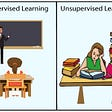# Understanding Naive Bayes Theorem For Machine Learning

Naive Bayes is a supervised machine learning algorithm. We are applying Bayes Theorem to do the classification.

So, Bayes theorem gives the probability of the relationship, given class variable ‘y’ and dependent feature vector (from X1 to Xn).

So to understand this, we take an example of ‘wrenches’

There are 2 machines that are creating wrenches .

So, We compile wrenches from 2 machines(1 & 2) and see that there are some wrenches that are defected.

Now, we need to find the probability that we randomly take the wrench from the above pile, such that it has defected and it is from machine 2.

Now Bayes Theorem comes into play, where we have to approach stepwise.

Step 1. We are having a total of 50 wrenches and from that 30 from machine1 and 20 from machine 2.

Step 2. We need to consider some prerequisite that is the defect is 1%

Step 3. Out of all the defective parts, we need to find out the probability from machine 1 and machine 2 that is 50% from machine 1 and 50% from machine 50%.

Step 4. Now looking into the problem, What is the probability that a wrench produced by machine2 is defective?

According to Bayes Theorem:

Compiling the values :

Putting the above values into our Bayes theorem formula:

Summary: So as per the problem we got the probability of 1.25%.

Now, we take a real-life example where the problem is that in order to reach a designation person having a particular ‘age’ and ‘salary’ as a feature.

Now the problem is, we enter a new data point that using Bayes theorem we have to find the probability that is a person having features ‘salary’ and ‘age’ choose to walk to reach a particular designation?

According to Bayes Theorem,

Step 1. Find Prior Probability that is P(Walks)?

Step 2. Find Marginal Likelihood that is P(X)?

For this, we need to create a local circle having similar features as of new points, randomly choose the radius of the local circle.

Step 3. Find the likelihood?

Likelihood of people who walks but having the given features, ‘age’ and ‘salary’.

Now, putting the values according to Bayes Theorem,

Similarly, we can find the probability that is a person having features ‘salary’ and ‘age’ choose to drive to reach a particular designation?

And the result is,

Hence, comparing the results we can say that a particular person chooses to walk rather than drive as the probability of the walk is 75% as compared to drive probability that is 25%.

Now, we will do the implementation part. We first import our data set of people who want to buy a specific product.

We should follow the steps to build a Naive Bayes Algorithm.

Step 1. Import the Libraries

Step 2. Importing the Dataset

Step 3. Split the data into a matrix of features(X)(So we are taking ‘Age’ and ‘Salary’ into consideration to do Prediction) and the dependent variable(y).

Step 4. Splitting the matrix of features(X) and dependent variable(y) into training and test set.

Steps 5. Now we do Feature Scaling for ‘Age’ and ‘Salary’ column.

Step 6. Fitting a linear model to test and training dataset.

Step 7. Predicting the Test result.

Step 8. Making the Confusion Matrix to do predictions.

Step 8. Visualization of Dataset.

--

--

--

## More from Manik Soni

Entrepreneur

Love podcasts or audiobooks? Learn on the go with our new app.

## Parle and Gradient Descent for UI Layouts## Machine Learning vs Deep Learning: Here’s what you must know!## When You Want Holt-Winters Instead of Machine Learning## Eligibility Traces in Reinforcement Learning## Supervised Learning and Linear Regression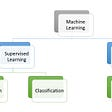## Accelerate PyTorch Model With TensorRT via ONNX## Max Pooling in Convolutional Neural Network(CNN)## Deep Learning for Graphs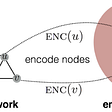Entrepreneur

## Beginner Machine Learning: 2) Multiple Linear Regression in Python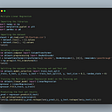## Learning journey 08/02/2022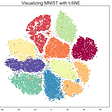## All About Confusion Matrix in Machine Learning — Theory & Code## Supervised and Unsupervised Machine Learning Algorithms